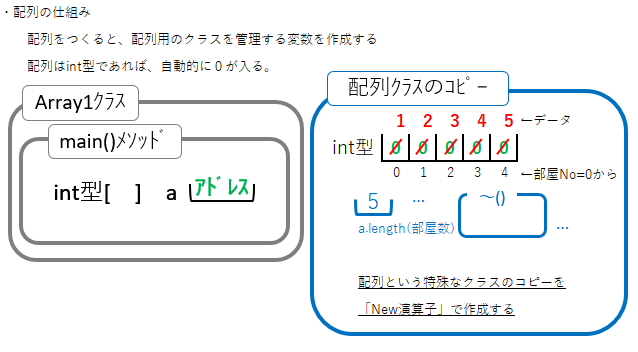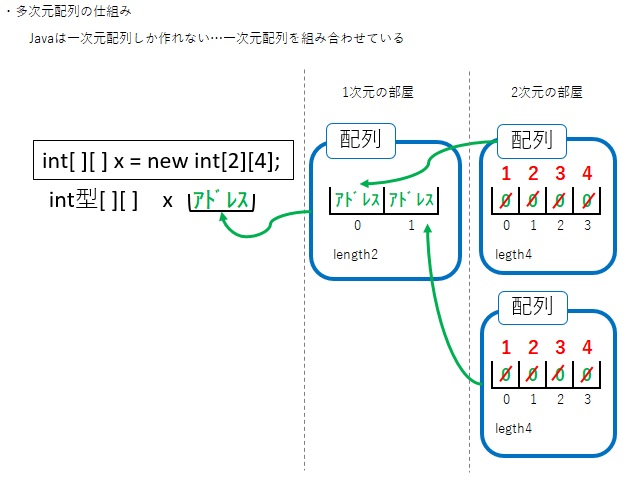# Array

An array is an expression that prepares a box for storing data side by side and puts it in and out. There are two types of arrays: one-dimensional arrays and multidimensional arrays. I will explain the mechanism of the array using an example.

# One-dimensional array

Let's substitute 1,2,3,4,5 for each element of the array and display it.

#### `Array1`

``````

class Array {
public static void main(String[] args) {

int[] a = new int;

for (int i = 0; i < a.length; i++){
a[i] = i + 1;
}

for (int i = 0; i < a.length; i++){
System.out.println("a[" + i + "] = " + a[i]);
}
}
}
``````
``````【Execution result】
a=1
a=2
a=3
a=4
a=5
``````# Multidimensional array

Let's substitute 1,2,3,4,1,2,3,4 for each element of the array and display it.

#### `Array2`

``````
class A2 {
public static void main(String[] args) {

int[][] x = new int;

for (int i = 0; i < 2; i++) {
for (int j = 0; j < 4; j++) {
x[i][j] = j + 1;
System.out.println("x[" + i + "][" + j + "] = " + x[i][j]);
}
}
}
}
``````
``````【Execution result】
x = 1
x = 2
x = 3
x = 4
x = 1
x = 2
x = 3
x = 4
``````